#### Yaw, pitch, and roll rotations

A 3D body can be rotated about three orthogonal axes, as shown in Figure 3.8. Borrowing aviation terminology, these rotations will be referred to as yaw, pitch, and roll:

1. A yaw is a counterclockwise rotation of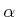about the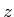-axis. The rotation matrix is given by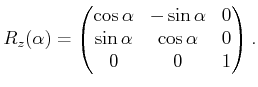(3.39)

Note that the upper left entries of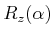form a 2D rotation applied to theand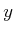coordinates, whereas thecoordinate remains constant.

2. A pitch is a counterclockwise rotation of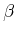about the-axis. The rotation matrix is given by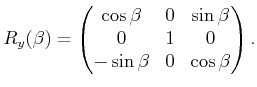(3.40)

3. A roll is a counterclockwise rotation of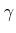about the-axis. The rotation matrix is given by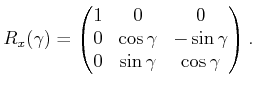(3.41)

Each rotation matrix is a simple extension of the 2D rotation matrix, (3.31). For example, the yaw matrix,, essentially performs a 2D rotation with respect to theandcoordinates while leaving thecoordinate unchanged. Thus, the third row and third column oflook like part of the identity matrix, while the upper right portion oflooks like the 2D rotation matrix.

The yaw, pitch, and roll rotations can be used to place a 3D body in any orientation. A single rotation matrix can be formed by multiplying the yaw, pitch, and roll rotation matrices to obtain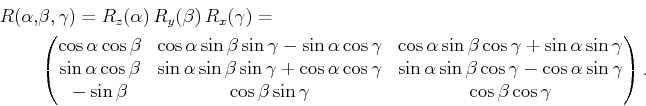(3.42)

It is important to note that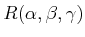performs the roll first, then the pitch, and finally the yaw. If the order of these operations is changed, a different rotation matrix would result. Be careful when interpreting the rotations. Consider the final rotation, a yaw by. Imagine sitting inside of a robot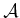that looks like an aircraft. If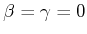, then the yaw turns the plane in a way that feels like turning a car to the left. However, for arbitrary values ofand, the final rotation axis will not be vertically aligned with the aircraft because the aircraft is left in an unusual orientation beforeis applied. The yaw rotation occurs about the-axis of the world frame, not the body frame of. Each time a new rotation matrix is introduced from the left, it has no concern for original body frame of. It simply rotates every point in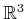in terms of the world frame. Note that 3D rotations depend on three parameters,,, and, whereas 2D rotations depend only on a single parameter,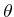. The primitives of the model can be transformed using, resulting in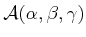.

Steven M LaValle 2020-08-14